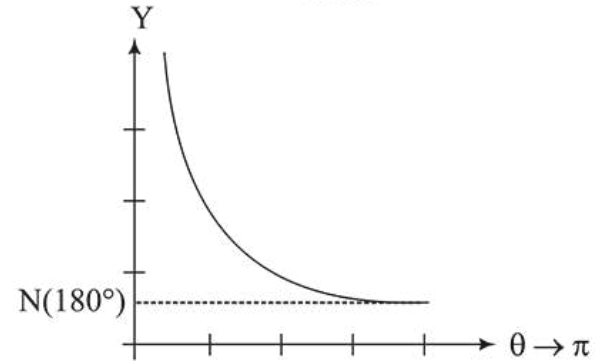# The graph which depicts the results of Rutherford gold foil

Question:

The graph which depicts the results of Rutherford gold foil

experiment with

$\alpha$-particles is:

$\theta$ : Scattering angle

$Y$ : Number of scattered $\alpha$-particles detected

(Plots are schematic and not to scale)

1. (1)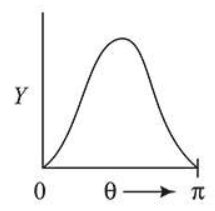2. (2)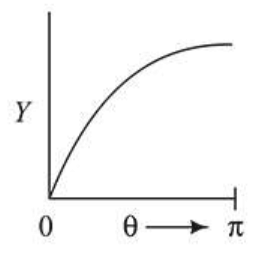3. (3)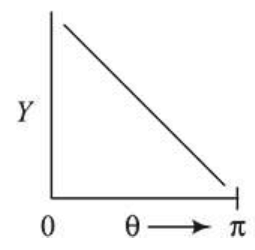4. (4)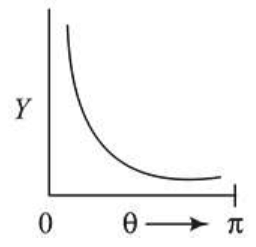Correct Option: , 4

Solution:

(4

)As per Rutherford $\alpha$-particle scattering experiment, number of $\alpha$-particles scattered $(N)$ related with deflection angle $\theta$ as

$N \propto \frac{1}{\sin ^{4} \frac{\theta}{2}}$ i.e. $Y \propto \frac{1}{\sin ^{4}\left(\frac{\theta}{2}\right)}$

$\therefore$ Corresponding $Y_{\text {versus }} \theta$ graph is as shown below.